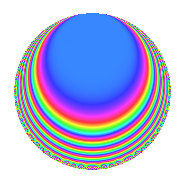# Properties

 Label 800.1.pLevel 800 Weight 1 Character orbit p Rep. character $$\chi_{800}(193,\cdot)$$ Character field $$\Q(\zeta_{4})$$ Dimension 6 Newform subspaces 3 Sturm bound 120 Trace bound 3

# Related objects

## Defining parameters

 Level: $$N$$ = $$800 = 2^{5} \cdot 5^{2}$$ Weight: $$k$$ = $$1$$ Character orbit: $$[\chi]$$ = 800.p (of order $$4$$ and degree $$2$$) Character conductor: $$\operatorname{cond}(\chi)$$ = $$5$$ Character field: $$\Q(i)$$ Newform subspaces: $$3$$ Sturm bound: $$120$$ Trace bound: $$3$$

## Dimensions

The following table gives the dimensions of various subspaces of $$M_{1}(800, [\chi])$$.

Total New Old
Modular forms 58 6 52
Cusp forms 10 6 4
Eisenstein series 48 0 48

The following table gives the dimensions of subspaces with specified projective image type.

$$D_n$$ $$A_4$$ $$S_4$$ $$A_5$$
Dimension 6 0 0 0

## Trace form

 $$6q + O(q^{10})$$ $$6q + 2q^{13} + 2q^{17} - 8q^{21} - 2q^{37} - 2q^{53} - 2q^{73} + 2q^{81} - 2q^{97} + O(q^{100})$$

## Decomposition of $$S_{1}^{\mathrm{new}}(800, [\chi])$$ into newform subspaces

Label Dim. $$A$$ Field Image CM RM Traces $q$-expansion
$$a_2$$ $$a_3$$ $$a_5$$ $$a_7$$
800.1.p.a $$2$$ $$0.399$$ $$\Q(\sqrt{-1})$$ $$D_{4}$$ $$\Q(\sqrt{-5})$$ None $$0$$ $$-2$$ $$0$$ $$2$$ $$q+(-1+i)q^{3}+(1+i)q^{7}-iq^{9}-q^{21}+\cdots$$
800.1.p.b $$2$$ $$0.399$$ $$\Q(\sqrt{-1})$$ $$D_{4}$$ $$\Q(\sqrt{-1})$$ None $$0$$ $$0$$ $$0$$ $$0$$ $$q+iq^{9}+(1-i)q^{13}+(1+i)q^{17}+(-1+\cdots)q^{37}+\cdots$$
800.1.p.c $$2$$ $$0.399$$ $$\Q(\sqrt{-1})$$ $$D_{4}$$ $$\Q(\sqrt{-5})$$ None $$0$$ $$2$$ $$0$$ $$-2$$ $$q+(1-i)q^{3}+(-1-i)q^{7}-iq^{9}-q^{21}+\cdots$$

## Decomposition of $$S_{1}^{\mathrm{old}}(800, [\chi])$$ into lower level spaces

$$S_{1}^{\mathrm{old}}(800, [\chi]) \cong$$ $$S_{1}^{\mathrm{new}}(160, [\chi])$$$$^{\oplus 2}$$

## Hecke Characteristic Polynomials

$p$ $F_p(T)$
$2$ ()()()
$3$ ($$( 1 + T )^{2}( 1 + T^{2} )$$)($$1 + T^{4}$$)($$( 1 - T )^{2}( 1 + T^{2} )$$)
$5$ ()()()
$7$ ($$( 1 - T )^{2}( 1 + T^{2} )$$)($$1 + T^{4}$$)($$( 1 + T )^{2}( 1 + T^{2} )$$)
$11$ ($$( 1 + T^{2} )^{2}$$)($$( 1 + T^{2} )^{2}$$)($$( 1 + T^{2} )^{2}$$)
$13$ ($$1 + T^{4}$$)($$( 1 - T )^{2}( 1 + T^{2} )$$)($$1 + T^{4}$$)
$17$ ($$1 + T^{4}$$)($$( 1 - T )^{2}( 1 + T^{2} )$$)($$1 + T^{4}$$)
$19$ ($$( 1 - T )^{2}( 1 + T )^{2}$$)($$( 1 - T )^{2}( 1 + T )^{2}$$)($$( 1 - T )^{2}( 1 + T )^{2}$$)
$23$ ($$( 1 + T )^{2}( 1 + T^{2} )$$)($$1 + T^{4}$$)($$( 1 - T )^{2}( 1 + T^{2} )$$)
$29$ ($$( 1 + T^{2} )^{2}$$)($$( 1 - T )^{2}( 1 + T )^{2}$$)($$( 1 + T^{2} )^{2}$$)
$31$ ($$( 1 + T^{2} )^{2}$$)($$( 1 + T^{2} )^{2}$$)($$( 1 + T^{2} )^{2}$$)
$37$ ($$1 + T^{4}$$)($$( 1 + T )^{2}( 1 + T^{2} )$$)($$1 + T^{4}$$)
$41$ ($$( 1 + T^{2} )^{2}$$)($$( 1 + T^{2} )^{2}$$)($$( 1 + T^{2} )^{2}$$)
$43$ ($$( 1 - T )^{2}( 1 + T^{2} )$$)($$1 + T^{4}$$)($$( 1 + T )^{2}( 1 + T^{2} )$$)
$47$ ($$( 1 + T )^{2}( 1 + T^{2} )$$)($$1 + T^{4}$$)($$( 1 - T )^{2}( 1 + T^{2} )$$)
$53$ ($$1 + T^{4}$$)($$( 1 + T )^{2}( 1 + T^{2} )$$)($$1 + T^{4}$$)
$59$ ($$( 1 - T )^{2}( 1 + T )^{2}$$)($$( 1 - T )^{2}( 1 + T )^{2}$$)($$( 1 - T )^{2}( 1 + T )^{2}$$)
$61$ ($$( 1 + T^{2} )^{2}$$)($$( 1 + T^{2} )^{2}$$)($$( 1 + T^{2} )^{2}$$)
$67$ ($$( 1 - T )^{2}( 1 + T^{2} )$$)($$1 + T^{4}$$)($$( 1 + T )^{2}( 1 + T^{2} )$$)
$71$ ($$( 1 + T^{2} )^{2}$$)($$( 1 + T^{2} )^{2}$$)($$( 1 + T^{2} )^{2}$$)
$73$ ($$1 + T^{4}$$)($$( 1 + T )^{2}( 1 + T^{2} )$$)($$1 + T^{4}$$)
$79$ ($$( 1 - T )^{2}( 1 + T )^{2}$$)($$( 1 - T )^{2}( 1 + T )^{2}$$)($$( 1 - T )^{2}( 1 + T )^{2}$$)
$83$ ($$( 1 - T )^{2}( 1 + T^{2} )$$)($$1 + T^{4}$$)($$( 1 + T )^{2}( 1 + T^{2} )$$)
$89$ ($$( 1 + T^{2} )^{2}$$)($$( 1 - T )^{2}( 1 + T )^{2}$$)($$( 1 + T^{2} )^{2}$$)
$97$ ($$1 + T^{4}$$)($$( 1 + T )^{2}( 1 + T^{2} )$$)($$1 + T^{4}$$)